# Data Mining - (Decision) Rule

Some forms of predictive data mining generate rules that are conditions that imply a given outcome.

Rules are if-then-else expressions; they explain the decisions that lead to the prediction.

They are produced from a decision tree or association (such as association rule)

For example, a rule might specify that a person:

• who has a bachelor's degree (an attribute)
• and lives in a certain neighbourhood (an other attribute)

is likely to have an income greater than the regional average.

ODM Basket Analysis rules where antecedent and consequent contain the product ID.

## Type

### Classification

A classification rule predicts value of a given attribute:

````If outlook = sunny and humidity = high then play = no`
```

### Association

A Association rule predicts value of arbitrary attribute (or combination)

``````If temperature = cool                  then humidity = normal
If humidity = normal and windy = false then play = yes
If outlook = sunny and play = no       then humidity = high
If windy = false and play = no         then outlook = sunny and humidity = high```
```

## Set of Rules

rule is easy to interpret, but a complex set of rules probably isn’t.

A sequential cover algorithm for sets of rules with complex conditions. Sets of rules are hard to interpret.

## Algorithm

Strategies for Learning Each Rule:

• General-to-Specific:
• Add constraints to eliminate negative examples
• Stop when only positives are covered
• Specific-to-General
• Remove constraints in order to cover more positives
• Stop when further generalization results in covering negatives

If more than one rule is triggered (Conflicts),

• choose the “most specific” rule
• Use domain knowledge to order rules by priority

### General-to-Specific

``````Initialize the ruleset R to the empty set

for each class C {

while D is nonempty {

Construct one rule r that correctly classifies
some instances in D that belong to class C
and does not incorrectly classify any non-C instances
# See below "Finding next rule for class C"

Add rule r to ruleset R

Remove from D all instances correctly classified by r

}
}
return the ruleset R```
```

Sequential Covering: Finding next rule for class C

``````Initialize A as the set of all attributes over D

while r incorrectly classifies some non-C instances of D
{

write r as antecedent(r) => C

for each attribute-value pair (a=v),
where a belongs to A and v is a value of a,
compute the accuracy of the rule

antecedent(r) and (a=v) => C

let (a*=v*) be the attribute-value pair of
maximum accuracy over D; in case of a tie,
choose the pair that covers the greatest
number of instances of D

update r by adding (a*=v*) to its antecedent:
r = ( antecedent(r) and (a*=v*) ) => C
remove the attribute a* from the set A:
A = A - {a*}
}```
```

## Properties

### Support

Rules have an associated support (What percentage of the population satisfies the rule?).

### Confidence

Confidence is the proportion of the occurrences of the antecedent that result in the consequent e.g. how many times do we get C when we have A and B {A, B} ⇒ C

### Lift

Lift indicates the strength of a rule over the random co-occurrence of the antecedent and the consequent

## Documentation / Reference

Discover More(Function | Operator | Map | Mapping | Transformation | Method | Rule | Task | Subroutine)

Section computable function. A function is a callable unit that may be called: a procedure, a subrontine a routine, a method (belong to an objectmacrocomputablalgorithreusable blocargumentdevelopment...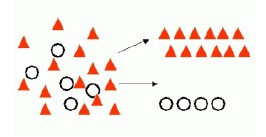Data Mining - (Classifier|Classification Function)

A classifier is a Supervised function (machine learning tool) where the learned (target) attribute is categorical (“nominal”) in order to classify. It is used after the learning process to classify...Data Mining - (Function|Model)

The model is the function, equation, algorithm that predicts an outcome value from one of several predictors. During the training process, the models are build. A model uses a logic and one of several...Data Mining - Apriori algorithm

Apriori is an Unsupervised Association algorithm performs market basket analysis by discovering co-occurring items (frequent itemsets) within a set. Apriori finds rules with support greater than a specified...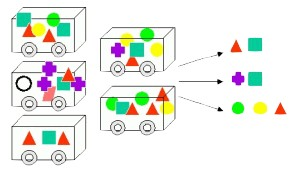Data Mining - Association (Rules Function|Model) - Market Basket Analysis

Association Rule is an unsupervised data mining function. It finds rules associated with frequently co-occurring items, used for: market basket analysis, cross-sell, and root cause analysis....Data Mining - Decision Tree (DT) Algorithm

Desicion Tree (DT) are supervised Classification algorithms. They are: easy to interpret (due to the tree structure) a boolean function (If each decision is binary ie false or true) Decision trees...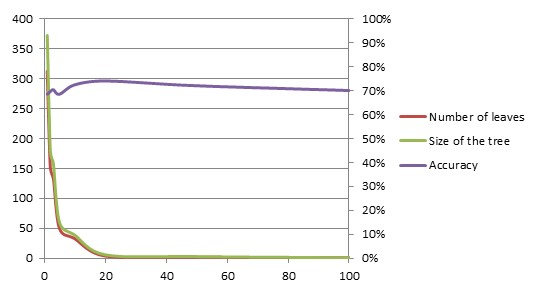Data Mining - Pruning (a decision tree, decision rules)

Pruning is a general technique to guard against overfitting and it can be applied to structures other than trees like decision rules. A decision tree is pruned to get (perhaps) a tree that generalize...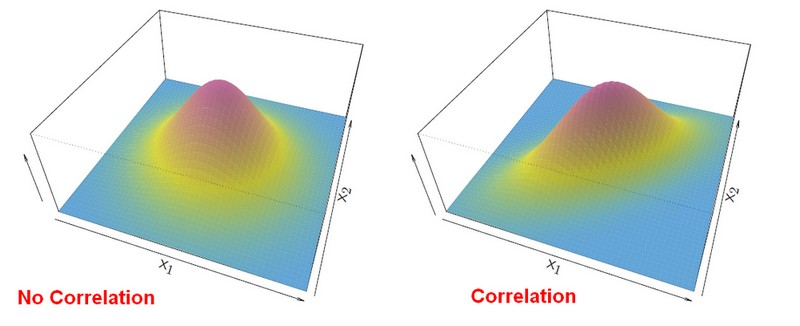Statistics - Fisher (Multiple Linear Discriminant Analysis|multi-variant Gaussian)

multi-variant Gaussians. Fisher has describe first this analysis with his Iris Data Set. A Fisher's linear discriminant analysis or Gaussian LDA measures which centroid from each class is the closest....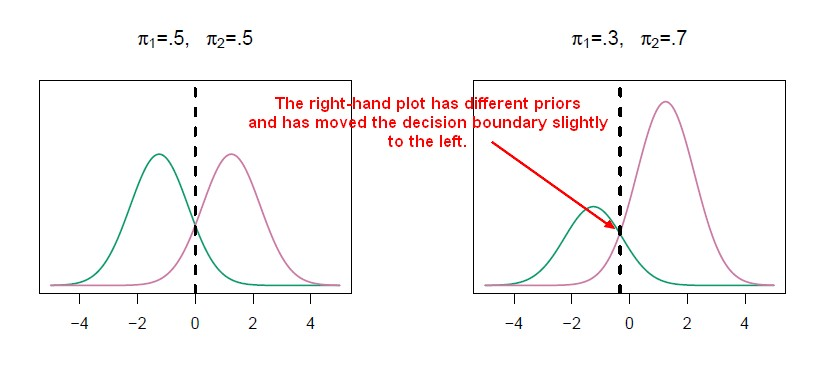Statistics Learning - Discriminant analysis

Discriminant analysis is a classification method. In discriminant analysis, the idea is to: model the distribution of X in each of the classes separately. use what's known as Bayes theorem to flip...